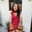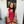Trusted answers to developer questions
Trusted Answers to Developer Questions

Related Tags

# What is the Feistel cipher structure?Aqsa Amir

Grokking Modern System Design Interview for Engineers & Managers

Ace your System Design Interview and take your career to the next level. Learn to handle the design of applications like Netflix, Quora, Facebook, Uber, and many more in a 45-min interview. Learn the RESHADED framework for architecting web-scale applications by determining requirements, constraints, and assumptions before diving into a step-by-step design process.

## Overview

Feistel cipher is a design model that derives different symmetric block ciphers, such as DESData Encryption Standard. It uses the same key for the encryption and decryption process.

Feistel cipher structure encrypts plain text in several rounds, where it applies substitutionManipulating the data using an encryption function. and permutationIt swaps the two halves of the current round to generate data block for the next round. to the data. Each round uses a different key for encryption, and that same key is used for the decryption process.

### Encryption

Feistel cipher structure converts plain text to cipher text using the following steps:

The Feistel cipher structure

### Steps

The Feistel cipher structure above follows the steps below:

1. Convert plain text into binary using ASCII codes of each character.
2. Divide the data into blocks, processed one at a time.
3. The encryption process takes two inputs, one block of data and a master key.
4. When the block is ready for the encryption process, divide it into two halves of equal length. The left half is denoted by $L_{0}$ and the right half is characterized by$R_{0}$.
5. Data is passed through $n$ rounds of execution, where the $n$ is specified by the design of the algorithm.
6. Each round uses the same encryption function and a different sub key generated from the master key.
7. To generate the left half of the next round, $L_{i+1}$, the current right half, $R_{i}$ is assigned to it.
8. To generate the right half of the next round, $R_{i+1}$, the current right half, $R_{i}$ undergoes the following steps:
1. $R_{i}$ and the $subkey_{i}$ is passed through an encryption function.
2. The result from step I is XORed with the left half of the current round, $L_{i}$.
3. The result from step II is assigned to the right half of the next round, $R_{i+1}$.
9. The left and right half of data obtained after n rounds of execution is swapped again before concluding the Feistel cipher.

### Decryption

The decryption process uses a similar procedure: cipher text is fed to the algorithm and the exact steps are followed. The only difference is that the keys used in the decryption process follow a reverse order of that used in the encryption process.

Note: Ensure that the same key is used for encryption and decryption process for the same rounds.

### Design features

Feistel cipher structure has the following five components:

• Number of rounds: The greater the number of rounds, $n$, used for the encryption/decryption process, the higher the complexity; hence, the security of the block cipher.
• Sub key generation algorithm: Complex algorithms make it difficult for intruders to crack the key.
• Encryption function: Complex functions enhance the security of the block cipher, making them difficult to crack.
• Block size: The larger the size of the block, the more secure and complex the block cipher is. However, a larger block size reduces the execution speed of the encryption and decryption process.
• Key size: A large size key increases the security of the block cipher. However, it also makes the encryption and decryption process slow.

RELATED TAGS

CONTRIBUTORAqsa Amir